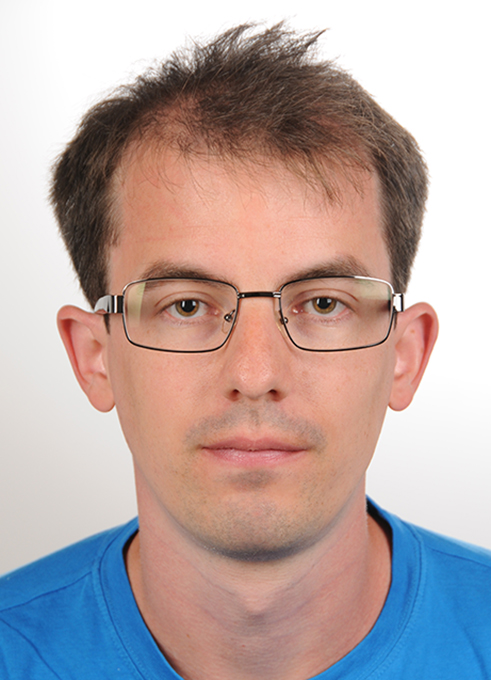Homepage of Tony Stillfjord Assistant professor (biträdande universitetslektor) at the Centre for Mathematical Sciences, Lund University, Sweden. Position funded by WASP. Previously: postdoc at the MPI Magdeburg, Germany. (CSC group) Contents: Contact information CV   Preprints   Publications   Thesis   Peer review   Software

Research
I am a numerical analyst; I construct, analyze and implement numerical methods for approximating the solutions to various problems, typically partial differential equations. Most of my work concerns different types of splitting schemes, which can be applied to a large number of problem classes to great effect. During the last few years, I have been working on large-scale (differential) Riccati equations, which are used in optimal control problems. Recently, I have also become interested in numerical methods for machine learning in the context of artificial intelligence.

Short CV
Born on February 13, 1986, in Myckleby, Sweden.

Education:
• Ph.D. in Numerical Analysis, Lund University, Lund, Sweden, 2015
• MSc. in Mathematics, Lund University, Lund, Sweden, 2010
• BSc. in Mathematics, Lund University, Lund, Sweden, 2009
• Assistant professor (biträdande universitetslektor), Lund University, Sweden, 2019-
• Postdoc, Max Planck Institute for Dynamics of Complex Technical Systems, Germany, 2017-2019
• Postdoc, Chalmers and the University of Gothenburg, Sweden, 2015-2017
1. with M. Eisenmann:
Sub-linear convergence of a tamed stochastic gradient descent method in Hilbert space
[preprint]
2. with M. Eisenmann, M. Williamson:
Sub-linear convergence for a stochastic proximal iteration method in Hilbert space
[preprint]
3. Convergence analysis for the exponential Lie splitting scheme applied to the abstract differential Riccati equation
[preprint]

1. with P. Benner, C. Trautwein:
A linear implicit Euler method for the finite element discretization of a controlled stochastic heat equation
IMA J. Numer. Anal. (2021)
[journal]
2. with H. Mena, L.-M. Pfurtscheller:
GPU acceleration of splitting schemes applied to differential matrix equations
Numer. Algor. 83 (2020), pp. 395--419
[offprint] [journal]
3. Singular value decay of operator-valued differential Lyapunov and Riccati equations
SIAM J. Control Optim. 56(5) (2018), pp. 3598–3618
[offprint] [journal]
4. with A. Målqvist, A. Persson:
Multiscale differential Riccati equations for linear quadratic regulator problems
SIAM J. Sci. Comput. 40(4) (2018), pp. A2406–A2426
[offprint] [journal]
5. Adaptive high-order splitting schemes for large-scale differential Riccati equations
Numer. Algor. 78(4) (2018), pp. 1129–1151
[offprint] [journal]
6. with T. Damm, H. Mena:
Numerical Solution of the Finite Horizon Stochastic Linear Quadratic Control Problem
Numer. Linear Algebra Appl. 24(4) (2017), e2091
[preprint] [journal]
7. with A. Målqvist:
Finite element convergence analysis for the thermoviscoelastic Joule heating problem
BIT 57(3) (2017), pp.787-810
[preprint] [journal]
8. Low-rank second-order splitting of large-scale differential Riccati equations
IEEE Trans. Automat. Control 60(10) (2015), pp. 2791-2796
[preprint] [journal]
9. with E. Hansen:
Convergence analysis for splitting of the abstract differential Riccati equation
SIAM J. Numer. Anal. 52(6) (2014), pp. 3128-3139
[preprint] [journal]
10. with E. Hansen:
Implicit Euler and Lie splitting discretizations of nonlinear parabolic equations with delay
BIT 54(3) (2014), pp. 673-689
[preprint] [journal]
11. with E. Hansen:
Convergence of the implicit-explicit Euler scheme applied to perturbed dissipative evolution equations
Math. Comp. 82(284) (2013), pp. 1975-1985
[preprint] [journal]

• T. Stillfjord, Splitting schemes for nonlinear parabolic problems, 2015, LUP.

In addition to the LUP link, the thesis can be found here (with hyperlinks) or here (without hyperlinks). Both versions omit the included papers. For these, see above.

Peer review
I have refereed papers in the following journals:

• DREsplit
MATLAB-code for approximating the solution to a differential Riccati equation, based on the methods described in the papers 4 and 7 (listed above), is available here: DREsplit. This newest version unifies the interface for the different solvers and reduces code duplication.

Last updated on: 2021-02-15

Previous versions:

• BST20_CODE
The code which was used to run the experiments in the preprint listed as number 2 above is available here: BST20_CODE.